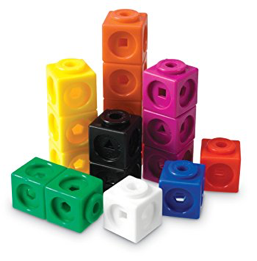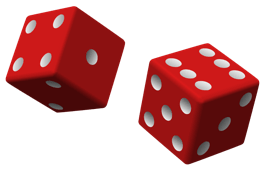# Problems to Ponder (October edition)

Welcome to this month’s edition of Problems to Ponder! Have an interesting solution? Send it to thevariable@smts.ca for publication in a future issue of The Variable!

Building Skyscrapers of Different Heights 

Stack 10 blocks (or linking cubes) to make any number of towers. The heights of the towers must all be different. Can you find all of the solutions?Adaptations and extensions: Stack 6 blocks to make towers of different heights. Stack 15 blocks to make towers of different heights. Find all of the solutions.

Get to Zero 

This game, played in teams of 2 or 3, provides students practice subtracting from 999. Students should be encouraged to check each players work and provide feedback for mistakes.

1. First, on a sheet of paper, each player writes the players’ names and the number 999 under them.
2. A player rolls three dice, then arranges the three numbers (for example, 2, 3, 5) in some order (for example, 235, 352, 532, and so on) and subtracts that 3-digit number from 999.
3. The players take turns, rolling the die to make a number and subtracting that number from their total.
4. The winner is the first player to reach 0, but they must get to 0 exactly.
5. At any time, a player may choose to roll only one or two dice, instead of three dice. If the only numbers a player can make are larger that his remaining score, the player loses his turn.

Ice Cream ScoopIn shops with lots of ice-cream flavors there are many different flavor combinations, even with only a 2-scoop cone.  With 1 ice-cream flavor there is 1 kind of 2-scoop ice cream, but with 2 flavors there are 3 possible combinations (eg vanilla/vanilla, chocolate/chocolate, and vanilla/chocolate).

Extensions:

• How many kinds of 2-scoop cones are there with 3 flavors? 4 flavors? 5 flavors?
• Explain how you would find the number of combinations for any number of flavors.

I Win!Two players each roll an ordinary six-sided die. Of the two numbers showing, the smaller is subtractedfrom the larger. If the difference is 0, 1, or 2, Player A gets 1 point. If the difference is 3, 4, or 5, Player B gets 1 point. The game ends after 12 rounds. The palyer with the most points wins the game. Is this game fair?

Extensions:

• Can you change the rules of the game so that it is fair?
• Roll two standard six-sided dice and divide the larger number showing by the smaller number. Player A gets 1 point if there is a remainder other than 0. Player B gets 1 point if the remainder is 0. Is this a fair game? If not, how would you make it fair?
• Assign numbers to the faces of the two dice so that, on any one roll if you add the numbers on the top faces, each sum from 1 to 12 is equally likely.

Cut the CakeYou and five friends want to share a 9-inch square chocolate cake with marshmallow icing. How can you cut the cake so that each person receives an equal share of both cake and icing? (Don’t forget the icing on the sides!)

Extensions:

• How would you share a 9-inch square cake among 5 people so that each person receives an equal share of both cake and icing?
• In how many different ways can you divide a rectangle into two pieces of the same size and shape?

Sources